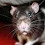## Monday, April 17, 2017

### N is for N-1

In my post on descriptive statistics, I introduced you to standard deviation, a measure of variability. Measures of variability are very important. First, we want to know how spread out a variable is (or variables are, when we're examining more than one). Second, we conduct statistical analyses to explain variance - to move variation from our error column into our systematic variation column; we need that information from standard deviation and its counterpart, variance, to have some variation to work with. Third, standard deviation and variance tell us something about how much scores will vary by chance alone.

Let's go back to our caffeine study example. I have two groups - experimental (which consumes regular coffee) and control (which consumes decaf). They have their coffee, then they take a test. The difference in mean test score between the two groups tells us how much variation is due to caffeine, while the standard deviation for each group tells us how much test scores will vary within a treatment group - that is, by chance alone. Not everyone who consumes coffee is going to get the same test score, nor will everyone who consumed decaf get the same score. Both groups are going to vary naturally, and until we know the particular reason they vary, we call that variation error (or variation by chance alone). If the difference between the two groups is larger than the differences within the groups, we conclude that caffeine was the cause of the difference.

But any time we conduct research, we're using a sample to represent a population. We're not interested in whether caffeine causes differences in test performance in our small sample alone. We want to draw larger conclusions - that caffeine is a way to improve test performance. We want to generalize those findings. So we draw a sample from the population and use the statistics we generate to represent that population.

The thing about samples is they don't always look like the population. In fact, if the variable we're interested in is normally distributed, we're likely to get a lot more people (proportionally) who fall in the middle of the distribution - because there are more of them - than people who fall in the tails.

That's because the y-axis (the height of the curve) represents frequency. There are a lot more people in the middle, so that's who we're more likely to get in our sample. Because of that, our sample distribution won't be as spread out as the population distribution, and our standard deviation is likely to underestimate the true population standard deviation. So, when we compute standard deviation for a sample (rather than a population), we apply a correction to bump the standard deviation up.

Standard deviation is essentially an average, but rather than being an average of the scores (the mean), it is the average amount scores deviate from the mean. Whenever we compute an average of some kind, we divide by the number of values (which we represent as N). To correct standard deviation for its underestimation, we divide instead by N-1. Now the sum of those deviations is divided into slightly fewer parts, resulting in a higher value.

If you took statistics, you probably first learned to compute standard deviation with N, and then suddenly, your professor said, "Cool, now that you understand standard deviation, I hate to break it to you but the proper formula is N-1." It's quite frustrating. I've taught stats a few times and my students are always thrown off by this sudden change of formula. Frankly, I think we should only teach it the second way - after all, it's highly unlikely you will actually be working with data from an entire population, unless you end up working for large survey firms or the Census Bureau - and then, teach about samples versus population standard deviation as more advanced topic later on in the course. After all, population values aren't even called statistics; that term is reserved for sample values. Population values are called parameters. But I digress.

#### 1 comment:

1.This is really interesting stuff. I've never really studied statistics.

Cait @ Click's Clan